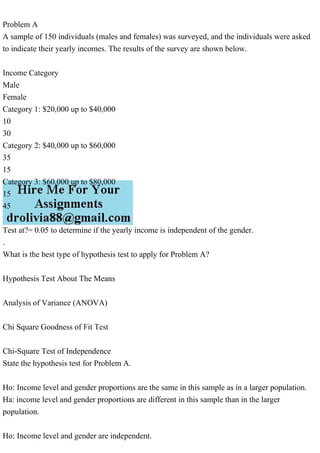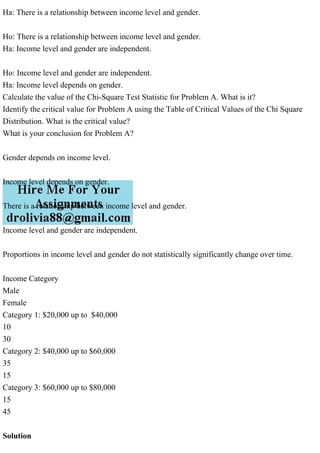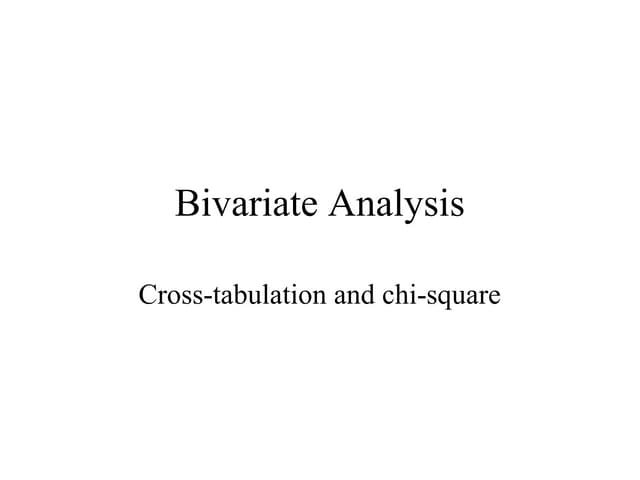Publicité

# Problem Axmlnamespace prefix = o ns = urnschemas-microsoft-com.pdf

ajantaopt
26 Mar 2023Prochain SlideShareBivariate analysis
Chargement dans ... 3
1 sur 3
Publicité

### Problem Axmlnamespace prefix = o ns = urnschemas-microsoft-com.pdf

1. Problem A A sample of 150 individuals (males and females) was surveyed, and the individuals were asked to indicate their yearly incomes. The results of the survey are shown below. Income Category Male Female Category 1: \$20,000 up to \$40,000 10 30 Category 2: \$40,000 up to \$60,000 35 15 Category 3: \$60,000 up to \$80,000 15 45 Test at?= 0.05 to determine if the yearly income is independent of the gender. . What is the best type of hypothesis test to apply for Problem A? Hypothesis Test About The Means Analysis of Variance (ANOVA) Chi Square Goodness of Fit Test Chi-Square Test of Independence State the hypothesis test for Problem A. Ho: Income level and gender proportions are the same in this sample as in a larger population. Ha: income level and gender proportions are different in this sample than in the larger population. Ho: Income level and gender are independent.
2. Ha: There is a relationship between income level and gender. Ho: There is a relationship between income level and gender. Ha: Income level and gender are independent. Ho: Income level and gender are independent. Ha: Income level depends on gender. Calculate the value of the Chi-Square Test Statistic for Problem A. What is it? Identify the critical value for Problem A using the Table of Critical Values of the Chi Square Distribution. What is the critical value? What is your conclusion for Problem A? Gender depends on income level. Income level depends on gender. There is a relationship between income level and gender. Income level and gender are independent. Proportions in income level and gender do not statistically significantly change over time. Income Category Male Female Category 1: \$20,000 up to \$40,000 10 30 Category 2: \$40,000 up to \$60,000 35 15 Category 3: \$60,000 up to \$80,000 15 45 Solution
3. in it the test statistic=28.125 Chi-Square Test of Independence Ho: There is a relationship between income level and gender. Income level depends on gender.
Publicité